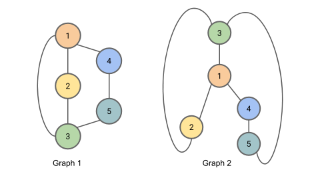# The Weisfeiler-Lehman Isomorphism Test

Resource | v1 | created by janarez |Type Blog post
Created unavailable
Identifier unavailable

Description

Two graphs are considered isomorphic if there is a mapping between the nodes of the graphs that preserves node adjacencies. Here is the algorithm for the Weisfeiler-Lehman Isomorphism Test. It produces for each graph a canonical form. If the canonical forms of two graphs are not equivalent, then the graphs are definitively not isomorphic. However, it is possible for two non-isomorphic graphs to share a canonical form, so this test alone cannot provide conclusive evidence that two graphs are isomorphic.

##### Relations

The graph isomorphism problem is the computational problem of determining whether two finite graphs a...7.0 /10
useless alright awesome
##### from 1 review
Tip: Rating is anonymous unless you also write a comment.
Resource level 2.0 /10